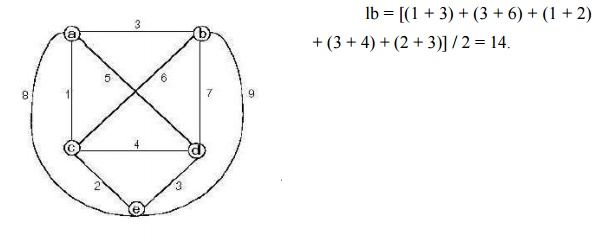Home | | Artificial Intelligence | Examples of search problems

# Examples of search problems

Traveling Salesman Problem: Given n cities with known distances between each pair, find the shortest tour that passes through all the cities exactly once before returning to the starting city.

Examples of search problems

Traveling Salesman Problem: Given n cities with known distances between each pair, find the shortest tour that passes through all the cities exactly once before returning to the starting city.

A lower bound on the length l of any tour can be computed as follows

For each city i, 1 â‰¤ i â‰¤ n, find the sum si of the distances from city i to the two nearest cities.

Compute the sum s of these n numbers.

Divide the result by 2 and round up the result to the nearest integer

lb = s / 2

The lower bound for the graph shown in the Fig 5.1 can be computed as follows:For any subset of tours that must include particular edges of a given graph, the lower bound can be modified accordingly. E.g.: For all the Hamiltonian circuits of the graph that must include edge (a, d), the lower bound can be computed as follows:

lb = [(1 + 5) + (3 + 6) + (1 + 2) + (3 + 5) + (2 + 3)] / 2 = 16.

Applying the branch-and-bound algorithm, with the bounding function lb = s / 2, to find the shortest Hamiltonian circuit for the given graph, we obtain the state-space tree as shown below:

To reduce the amount of potential work, we take advantage of the following two observations:

Since the graph is undirected, we can generate only tours in which b is visited before c.

In addition, after visiting n â€“ 1 cities, a tour has no choice but to visit the remaining unvisited city and return to the starting one is shown in the Fig 5.2Root node includes only the starting vertex a with a lower bound of lb = [(1 + 3) + (3 + 6) + (1 + 2) + (3 + 4) + (2 + 3)] / 2 = 14.

Node 1 represents the inclusion of edge (a, b)

lb = [(1 + 3) + (3 + 6) + (1 + 2) + (3 + 4) + (2 + 3)] / 2 = 14.

Node 2 represents the inclusion of edge (a, c). Since b is not visited before c, this node is terminated.

Node 3 represents the inclusion of edge (a, d)

lb = [(1 + 5) + (3 + 6) + (1 + 2) + (3 + 5) + (2 + 3)] / 2 = 16.

Node 1 represents the inclusion of edge (a, e)

lb = [(1 + 8) + (3 + 6) + (1 + 2) + (3 + 4) + (2 + 8)] / 2 = 19.

Among all the four live nodes of the root, node 1 has a better lower bound. Hence we branch from node 1.

Node 5 represents the inclusion of edge (b, c)

lb = [(1 + 3) + (3 + 6) + (1 + 6) + (3 + 4) + (2 + 3)] / 2 = 16.

Node 6 represents the inclusion of edge (b, d)

lb = [(1 + 3) + (3 + 7) + (1 + 2) + (3 + 7) + (2 + 3)] / 2 = 16.

Node 7 represents the inclusion of edge (b, e)

lb = [(1 + 3) + (3 + 9) + (1 + 2) + (3 + 4) + (2 + 9)] / 2 = 19.

Since nodes 5 and 6 both have the same lower bound, we branch out from each of them.

Node 8 represents the inclusion of the edges (c, d), (d, e) and (e, a). Hence, the length of the tour,

l = 3 + 6 + 4 + 3 + 8 = 24.

Node 9 represents the inclusion of the edges (c, e), (e, d) and (d, a). Hence, the length of the tour,

l = 3 + 6 + 2 + 3 + 5 = 19.

Node 10 represents the inclusion of the edges (d, c), (c, e) and (e, a). Hence, the length of the tour,

l = 3 + 7 + 4 + 2 + 8 = 24.

Node 11 represents the inclusion of the edges (d, e), (e, c) and (c, a). Hence, the length of the tour,

l = 3 + 7 + 3 + 2 + 1 = 16.

Node 11 represents an optimal tour since its tour length is better than or equal to the other live nodes, 8, 9, 10, 3 and 4.

The optimal tour is a â†’ b â†’ d â†’ e â†’ c â†’ a with a tour length of 16.

Study Material, Lecturing Notes, Assignment, Reference, Wiki description explanation, brief detail
Artificial Intelligence : Examples of search problems |